# 开发者实战 | 在英特尔开发者套件上用 OpenVINO™ 处理 U2-Net 模型的图像分割和背景替换

08/01 17:0001

02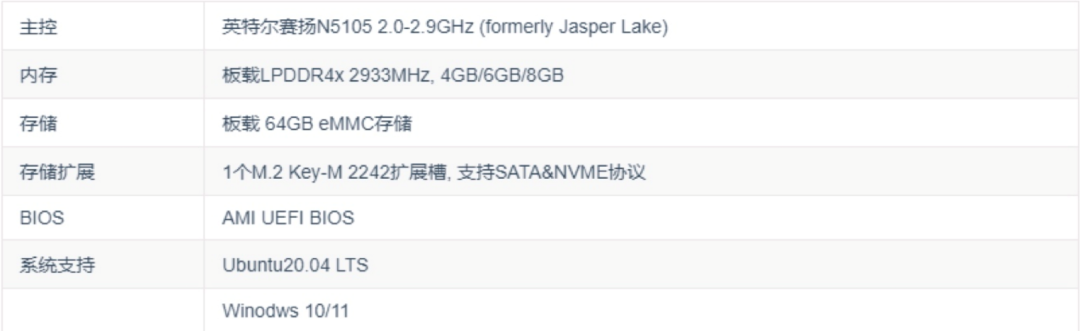03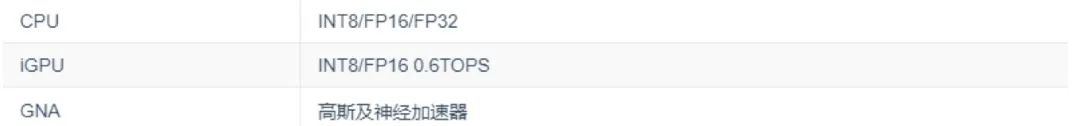04

• 开箱即用，不需要额外购买配件，不需要烧写系统，通电即用

• 性价比高，板载 8G 内存，64GB 存储，Wi-Fi6 千兆无线网卡，CPU + iGPU 异构计算

• 通用性好，流畅运行 Win10/Win11，桌面 Linux，软件兼容性好

• 易用性佳，提供丰富的案例资源，学习门槛低

• 官方认证，英特尔官方推荐开发套件，品质有保证

• 个性定制，可更换开机 Logo,产品个性化定制

05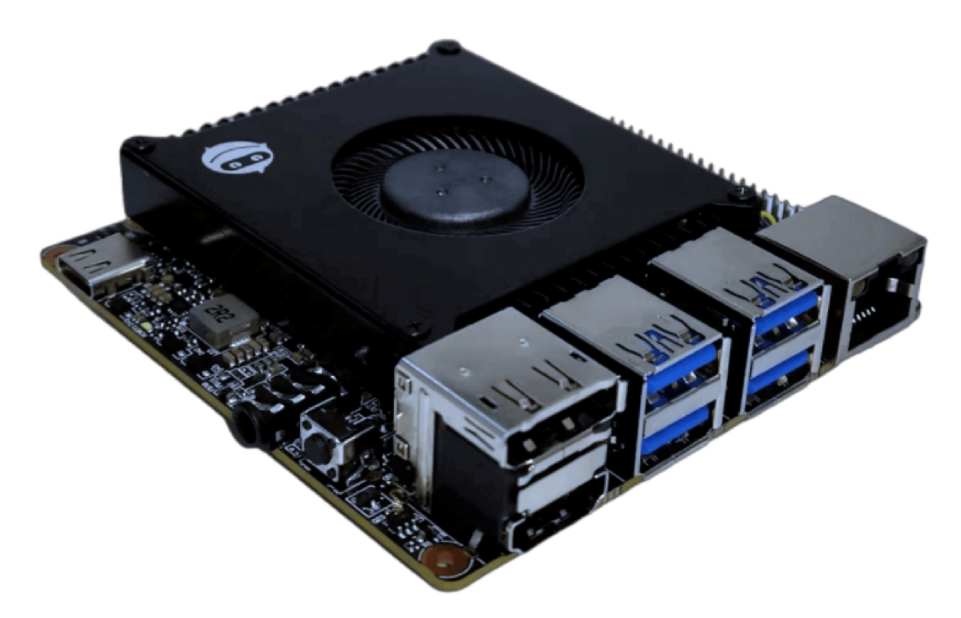01

https://mirrors.tuna.tsinghua.edu.cn/anaconda/archive/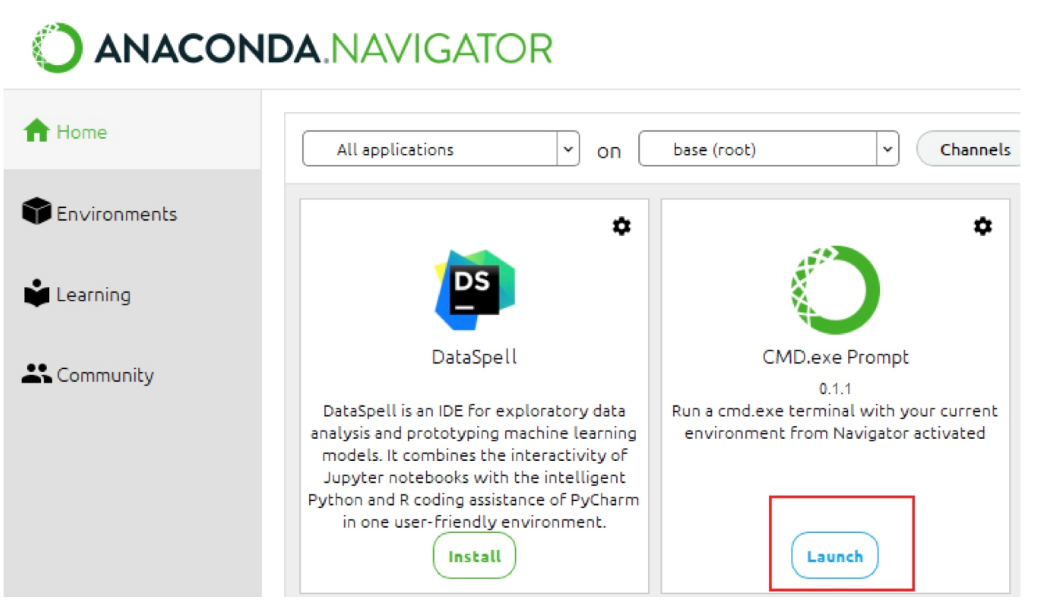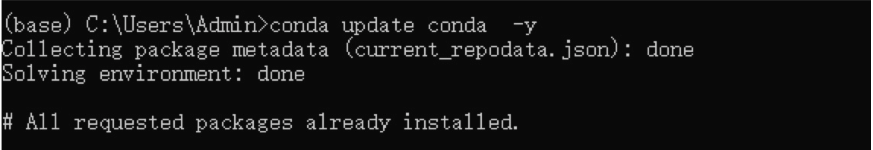pip更换清华源：

pip config set global.index-url https://pypi.tuna.tsinghua.edu.cn/simple02

pip install openvino-dev[ONNX,pytorch,tensorflow2]==2023.0.1

03

benchmark_app --help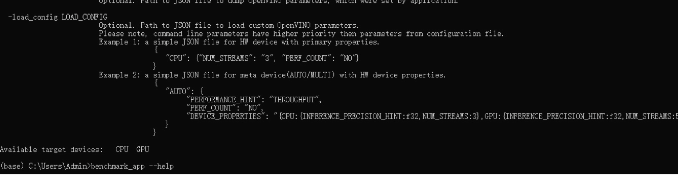U2-Net 模型的图像分割和背景替换演示

git clone https://github.com/openvinotoolkit/openvino_notebooks.git

01

U2-Net 在 RSU 的基础上开发的，用于显著目标检测（SOD），解决了在保持高分辨率特征图的同时，以较低的内存和计算成本使网络变得更深。U2 网络是一个两层嵌套的U型结构，如下图所示。它的 top-level 是一个由11个阶段组成的大U型结构（图中的立方体）。每一阶段都由一个配置良好的残差 Ublock（RSU）（bottom level  U结构）填充。因此，嵌套 U 结构能够更有效地提取阶段内多尺度特征和聚合阶段间多级特征。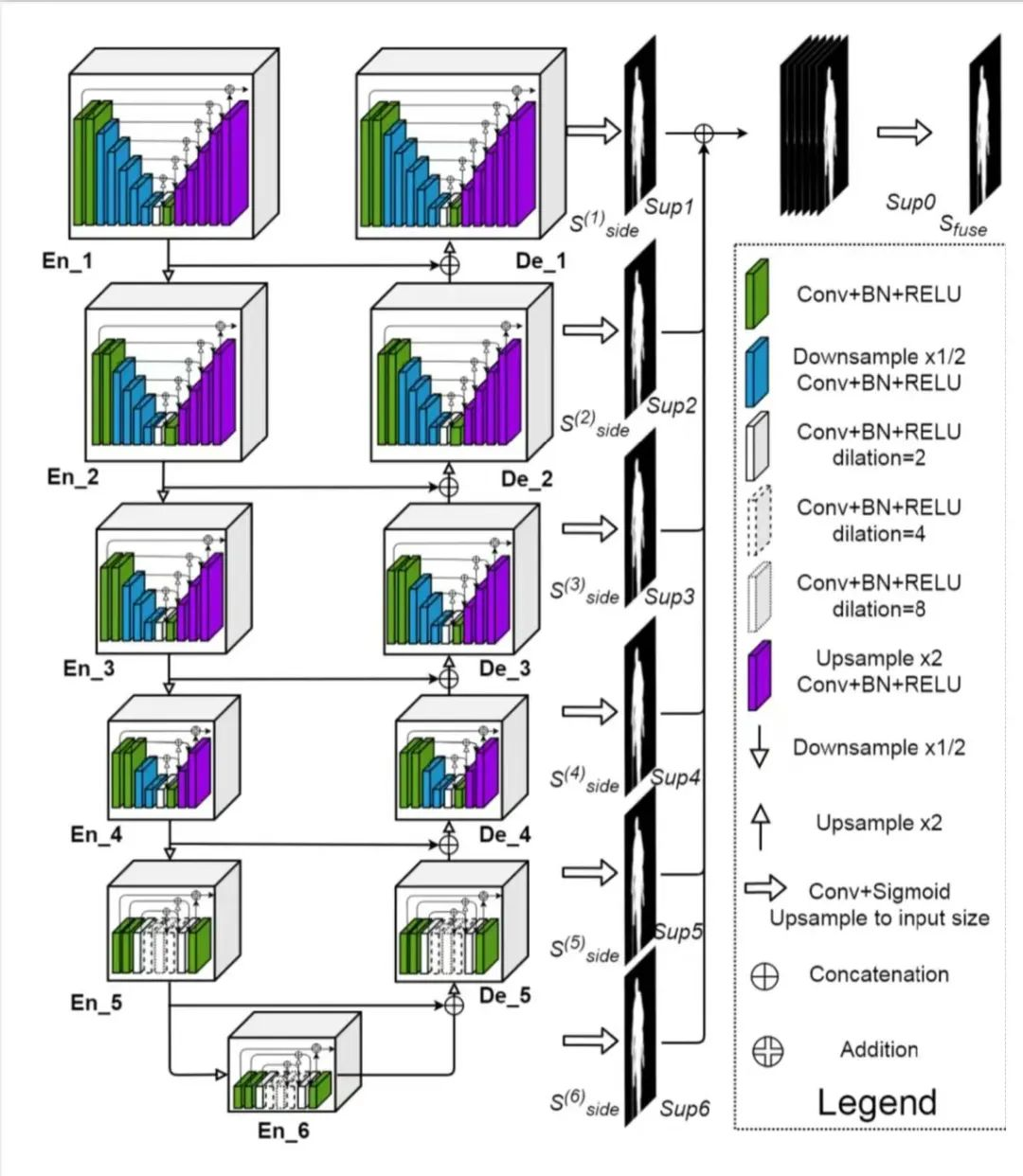02

u2net_lite = model_config(    name="u2net_lite",    url="https://drive.google.com/uc?id=1rbSTGKAE-MTxBYHd-51l2hMOQPT_7EPy",    model=U2NETP,    model_args=(),)model_path = Path(MODEL_DIR) / u2net_model.name / Path(u2net_model.name).with_suffix(".pth")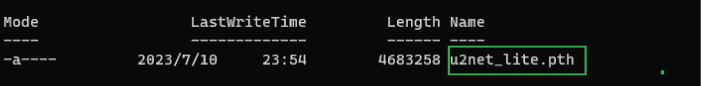03

# Load the model.net = u2net_model.model(*u2net_model.model_args)net.eval()# Load the weights.print(f"Loading model weights from: '{model_path}'")net.load_state_dict(state_dict=torch.load(model_path, map_location="cpu"))torch.onnx.export(net, torch.zeros((1,3,512,512)), "u2net.onnx")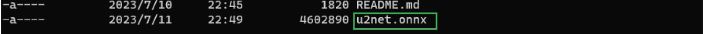04

model_ir = mo.convert_model(    "u2net.onnx",    mean_values=[123.675, 116.28 , 103.53],    scale_values=[58.395, 57.12 , 57.375],    compress_to_fp16=True

05

OpenVINO IR 模型需要 RGB 格式的图像。将图像转换为 RGB，将其大小调整为 512 x 512，并将尺寸转置为  OpenVINO IR 模型所需的格式。

IMAGE_URI = "https://storage.openvinotoolkit.org/repositories/openvino_notebooks/data/data/image/coco_hollywood.jpg"image = cv2.cvtColor(    src=load_image(IMAGE_URI),    code=cv2.COLOR_BGR2RGB,)resized_image = cv2.resize(src=image, dsize=(512, 512))input_image = np.expand_dims(np.transpose(resized_image, (2, 0, 1)), 0)

06

# Load the network to OpenVINO Runtime.ie = Core()compiled_model_ir = ie.compile_model(model=model_ir, device_name="CPU")# Get the names of input and output layers.input_layer_ir = compiled_model_ir.input(0)output_layer_ir = compiled_model_ir.output(0)# Do inference on the input image.start_time = time.perf_counter()result = compiled_model_ir([input_image])[output_layer_ir]end_time = time.perf_counter()print(    f"Inference finished. Inference time: {end_time-start_time:.3f} seconds, "    f"FPS: {1/(end_time-start_time):.2f}.")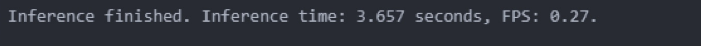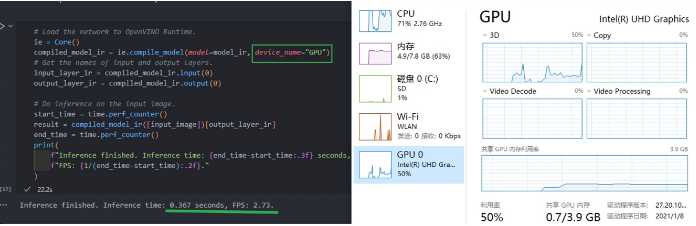07

resized_result = np.rint(    cv2.resize(src=np.squeeze(result), dsize=(image.shape, image.shape))).astype(np.uint8)# Create a copy of the image and set all background values to 255 (white).bg_removed_result = image.copy()bg_removed_result[resized_result == 0] = 255fig, ax = plt.subplots(nrows=1, ncols=3, figsize=(20, 7))ax.imshow(image)ax.imshow(resized_result, cmap="gray")ax.imshow(bg_removed_result)for a in ax:    a.axis("off")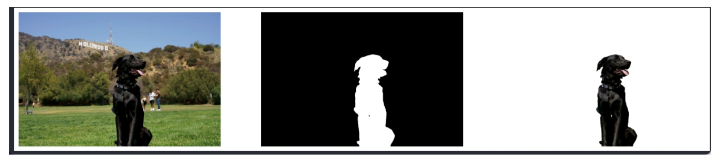08

background_image = cv2.cvtColor(src=load_image(BACKGROUND_FILE), code=cv2.COLOR_BGR2RGB)background_image = cv2.resize(src=background_image, dsize=(image.shape, image.shape))# Set all the foreground pixels from the result to 0# in the background image and add the image with the background removed.background_image[resized_result == 1] = 0new_image = background_image + bg_removed_result# Save the generated image.new_image_path = Path(f"{OUTPUT_DIR}/{Path(IMAGE_URI).stem}-{Path(BACKGROUND_FILE).stem}.jpg")cv2.imwrite(filename=str(new_image_path), img=cv2.cvtColor(new_image, cv2.COLOR_RGB2BGR))# Display the original image and the image with the new background side by sidefig, ax = plt.subplots(nrows=1, ncols=2, figsize=(18, 7))ax.imshow(image)ax.imshow(new_image)for a in ax:    a.axis("off")plt.show()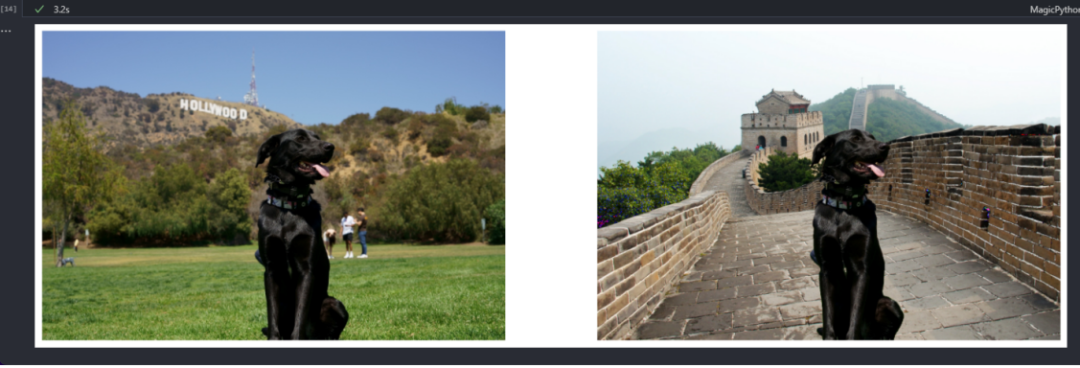--END--



你也许想了解(点击蓝字查看)⬇️

➡️ OpenVINO™ DevCon 2023重磅回归！英特尔以创新产品激发开发者无限潜能

➡️ 5周年更新 | OpenVINO™  2023.0，让AI部署和加速更容易

➡️ OpenVINO™5周年重头戏！2023.0版本持续升级AI部署和加速性能

➡️ OpenVINO™2023.0实战 | 在 LabVIEW 中部署 YOLOv8 目标检测模型

➡️ 开发者实战系列资源包来啦！

➡️

以AI作画，祝她节日快乐；简单三步，OpenVINO™ 助你轻松体验AIGC

➡️

还不知道如何用OpenVINO™作画？点击了解教程。

➡️

➡️

使用OpenVINO 在“端—边—云”快速实现高性能人工智能推理

➡️

图片提取文字很神奇？试试三步实现OCR！

➡️

【Notebook系列第六期】基于Pytorch预训练模型，实现语义分割任务

➡️

使用OpenVINO™ 预处理API进一步提升YOLOv5推理性能





扫描下方二维码立即体验
OpenVINO™ 工具套件 2023.0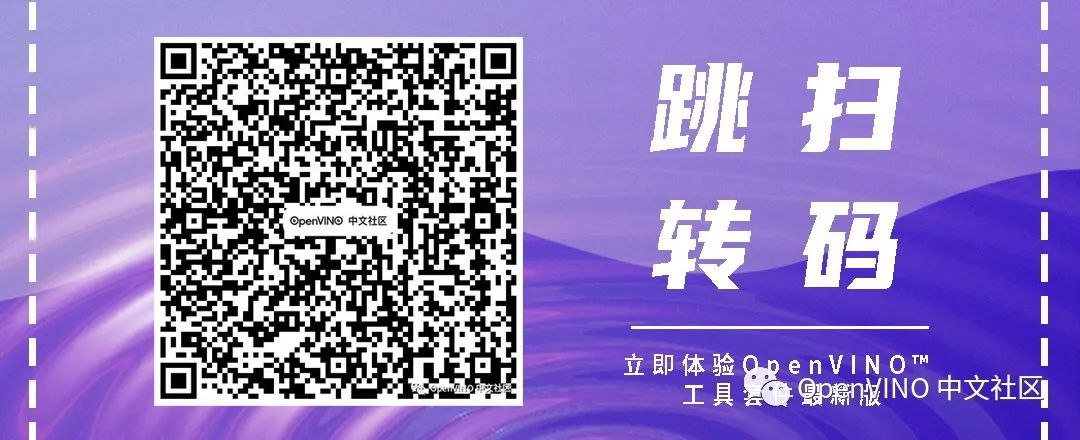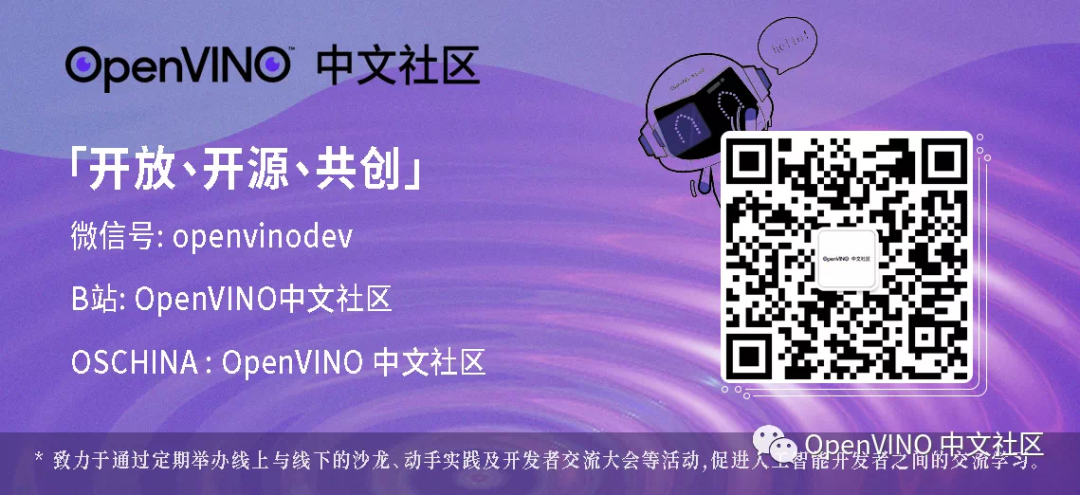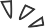0 评论
0 收藏
0